Courses

# 28 Year NEET Questions: Some Basic Principles And Techniques- 1

## 28 Questions MCQ Test Chemistry 31 Years NEET Chapterwise Solved Papers | 28 Year NEET Questions: Some Basic Principles And Techniques- 1

Description
This mock test of 28 Year NEET Questions: Some Basic Principles And Techniques- 1 for NEET helps you for every NEET entrance exam. This contains 28 Multiple Choice Questions for NEET 28 Year NEET Questions: Some Basic Principles And Techniques- 1 (mcq) to study with solutions a complete question bank. The solved questions answers in this 28 Year NEET Questions: Some Basic Principles And Techniques- 1 quiz give you a good mix of easy questions and tough questions. NEET students definitely take this 28 Year NEET Questions: Some Basic Principles And Techniques- 1 exercise for a better result in the exam. You can find other 28 Year NEET Questions: Some Basic Principles And Techniques- 1 extra questions, long questions & short questions for NEET on EduRev as well by searching above.
QUESTION: 1

### Which of the following compounds will exhibit cis-trans (geometrical) isomerism? 

Solution:

Alkenes with double bonds cannot undergo free rotation and can have different geometrical shapes with two different groups on each end of the double bond.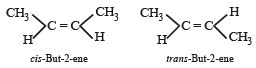QUESTION: 2

### Given are cycloh exanol (I) a cetic acid (II), 2, 4, 6 – trinitrophenol (III) and phenol (IV). In these the order of decreasing acidic character will be :

Solution: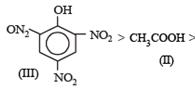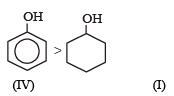Explanation: Presence of three — NO2 groups in o–, p– positions to phenolic groups (in III) makes phenol strongly acidic because its corresponding phenolate ion (conjugate base) is highly stabilized due to resonance.

Conjugate base of CH3COOH, (II) (i.e.CH3COO-) is a resonance hybrid of two equivalent structures.

The conjugate base of phenol, (IV) is stabilized due to resonance note that here all resonating structures are not equivalent).

The conjugate base of cyclohexanol, (I) does not exhibit resonance, hence not formed.

Hence A is the correct answer.

QUESTION: 3

### The correct order of increasing reactivity of C – X bond towards nucleophile in the following compounds is: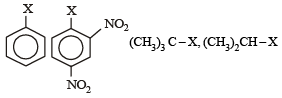(I)            (II)               (III)                    (IV)

Solution:

Tertiary alkyl halide is most reactive towards nucleophilic substitution because the corresponding carbocation (3°) is most stable. Aryl halide is least reactive due to partial double bond character of the C – Cl bond.

Presence of — NO2 groups in ortho and para positions  increases the reactivity of the – Cl towards nucleophiles.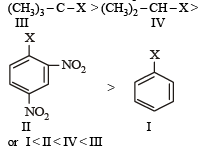QUESTION: 4

Among the given compounds , the most susceptible to nucleophilic attack at the carbonyl group is: 

Solution:

Cl is the weakest base and hence better leaving group

QUESTION: 5

Which one is most reactive towards electrophilic reagent? 

Solution: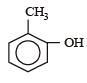Due to +M+M effect of −OH-OH group and hyperconjugation of −CH3-CH3 group.

QUESTION: 6

In the following the most stable conformation of n-butane is: 

Solution:

The bulky methyl groups are maximum away from each other.

QUESTION: 7

Which of the following conformers for ethylene glycol is most stable? 

Solution:

Due to hydrogen bonding between the two OH groups, gauche conformation of ethylene glycol (a) is the most stable conformation.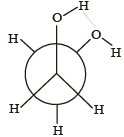QUESTION: 8

Which of the following species is not electrophilic in nature? 

Solution: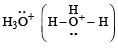has a lone pair of electrons on oxygen atom, thus not an electrophile. Also octet is complete.

QUESTION: 9

Which one of the following is most reactive towards electrophilic reagent ? 

Solution:

Due to +M effect of –OH group and hyperconjugation of –CH3 group.

Both methyl and hydroxyl groups are electron releasing groups hence +I effect increases. Therefore B will be most reactive in an electrophilic reaction.

Hence B is correct.

QUESTION: 10

The correct IUPAC name of the compound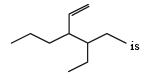Solution:

The given compound is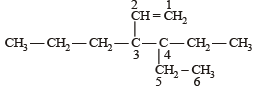4 ethyl, 3 propyl hex – 1 – ene

QUESTION: 11

The correct order of increasing bond length of C – H, C – O, C – C and C = C is : 

Solution:

Bond length order is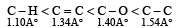QUESTION: 12

Which one is a nucleophilic substitution reaction among the following ? 

Solution:

Because of high electronegativities of the halogen atom, the carbon halogen (C – X) is highly polarised covalent bond. Thus, the carbon atom of the C – X bond becomes a good site for attack by nucleophiles (electron rich species). Nucleophilic substitution reactions are the most common reactions of alkyl halides.

QUESTION: 13

Which of the following compounds undergoes nucleophilic substitution reaction most easily ?

Solution:

In SNAr reactions, a carbanion is formed as an intermediate, so any substituent that increases the stability of carbanion and hence the transition state leading to its formation will enhance the SNAr reactions. To compare the rates of substitution in chlorobenzene, chlorobenzene having electron-withdrawing group, and chlorobenzene having electronreleasing group, we compare the structures carbanion I (from chlorobenzene), II (from chlorobenzene containing electron-withdrawing group) and III (from chlorobenzene containing electron-releasing group).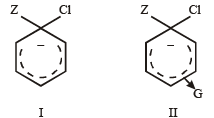G withdraws electrons, neutralises (disperses) –ve charge of the ring, stabilises carbanion, facilitates SN reaction (activation effect)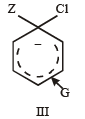G releases electrons, intensifies –ve charge, destabilizes carbanion, retards SN reaction (deactivation) NO2 is activating group and CH3 and OCH3 are deactiving group.
Hence, the correct order of nucleophilic substitution reactions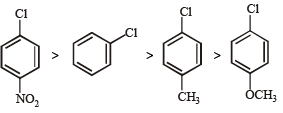QUESTION: 14

The IUPAC name of the following compound is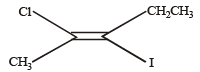Solution: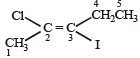Correct IUPAC name of above compound is trans-2-chloro-3-iodo-2-pentene

QUESTION: 15

In Duma's method of estimation of nitrogen 0.35 g of an organic compound gave 55 mL of nitrogen collected at 300 K temperature and 715 mm pressure. The percentage composition of nitrogen in the compound would be : (Aqueous tension at 300 K = 15 mm) 

Solution:

Given wt of compound taken (w) = 0.35 g
Volume of nitrogen collected (V) = 55 ml
Room temperature (t K) = 300 K
Atmospheric pressure (P) = 715 mm Aq. tension (ρ) = 15 mm
Calculation -Volume of N2 at NTP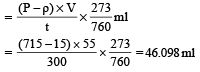% of nitrogen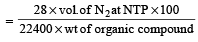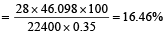QUESTION: 16

The Lassaigne’s extract is boiled with conc.HNO3 while testing for halogens. By doing so it 

Solution:

Conc. HNO3 decomposes NaCN and Na2S to avoid their interference.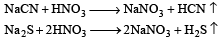QUESTION: 17

Among the following compounds the one that is most reactive towards electrophilic nitration is: 

Solution:

Electrophilic rate order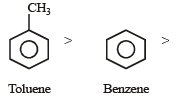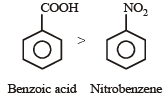Since nitration is an electrophilic substitution hence presence of electron releasing group like CH3 in the nucleus facilitates nitration.

QUESTION: 18

Which nomenclature is not according to IUPAC system? 

Solution: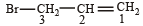The correct name is 3-Bromoprop-1-ene.

QUESTION: 19

Which of the following acids does not exhibit optical isomerism ? 

Solution: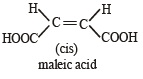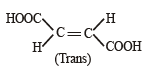It shows geometrical isomerism but does not show optical isomerism.

QUESTION: 20

Structure of the compound whose IUPAC name is 3-ethyl-2-hydroxy-4-methylhex-3-en-5-ynoic acid is : [NEET 2013]

Solution: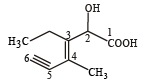IUPAC name of the structure is 3-ethyl-2-hydroxy-4-methylhex-3-en-5-ynoic acid

QUESTION: 21

Some meta-directing substituents in aromatic substitution are given. Which one is most deactivating? [NEET 2013]

Solution:

Decreasing order of deactivating effect of the given  m-directing group is > NO2 > – CN > – SO3H > – COOH
—NO2 group is most deactivating group due to strong – E, – I and – M effects.

QUESTION: 22

Which of the following compounds will not undergo Friedal-Craft’s reaction easily : [NEET 2013]

Solution:

–NO2 is a powerful electron withdrawing group. Its presence on ring makes the ring less active

QUESTION: 23

The structure of isobutyl group in an organic compound is : [NEET 2013]

Solution: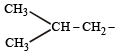(iso-butyl group)

QUESTION: 24

Arr ange the following in increasing order of stability [NEET Kar. 2013]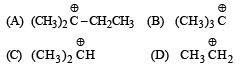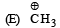Solution:

Stability depends on number of hyperconjugative structures.

QUESTION: 25

Given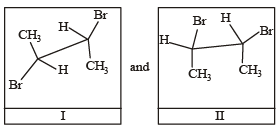I and II are [NEET Kar. 2013]

Solution:

Conformers are form of stereoisomers in which isomers can be interconverted by rotations about single bonds. I and II are staggered and eclipsed conformers respectively.

QUESTION: 26

Homolytic fission of the following alkanes forms free radicals CH3 – CH3, CH3 – CH2 – CH3, (CH3)2 CH – CH3, CH3 – CH2 – CH (CH3)2. Increasing order of stability of the radicals is [NEET Kar. 2013]

Solution:

Stability depends on number of hyperconjugative structure.

QUESTION: 27

What is the hybridisation state of benzyl carbonium   [NEET Kar. 2013]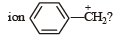Solution:

In the carbonium ion the carbon atom carrying the positive charge is sp2 hybridized.

QUESTION: 28

Nitrogen detection in an organic compound is carried out by Lassaigne’s test. The blue colour formed corresponds to which of the following formulae? [NEET Kar. 2013]

Solution:

The blue colour is of  Fe4 [Fe(CN)6]3